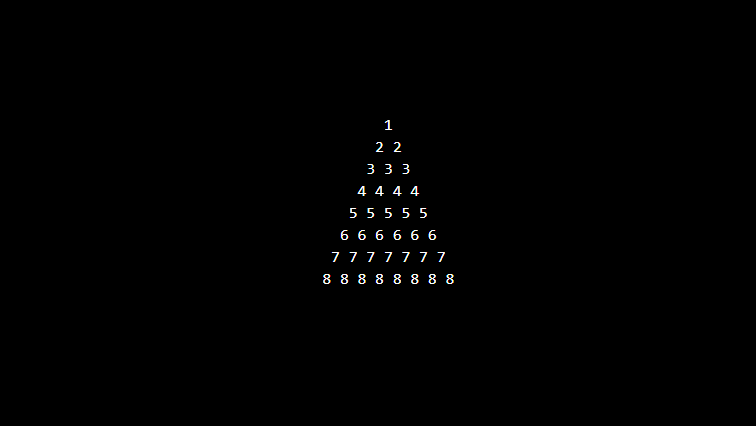In this article write a PHP program to print Diamond Triangle number. We run for loop 8 times, in which you 1 for loops which contains two nested for loops to print Diamond Triangle number as shown in the following program.

## Program to print Diamond Triangle number

```<?php
echo "<pre>";
for (\$i = 1; \$i <= 8; \$i++) {
for (\$k = 8; \$k >= \$i; \$k--) {
echo "&nbsp;";
}
for (\$j = 1; \$j <= \$i; \$j++) {
echo \$i . " ";
if (\$j == \$i) {
echo "&nbsp;";
echo "<br/>";
}
}
}
echo "</pre>";
?>
```

### Output: